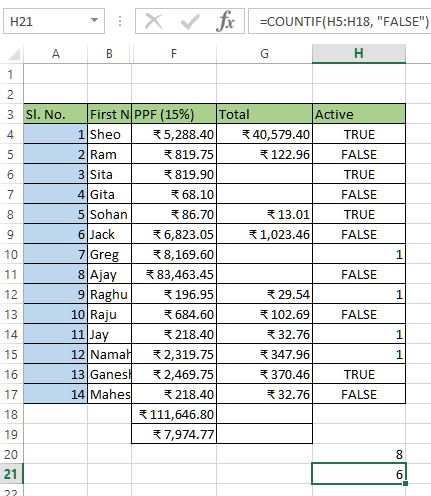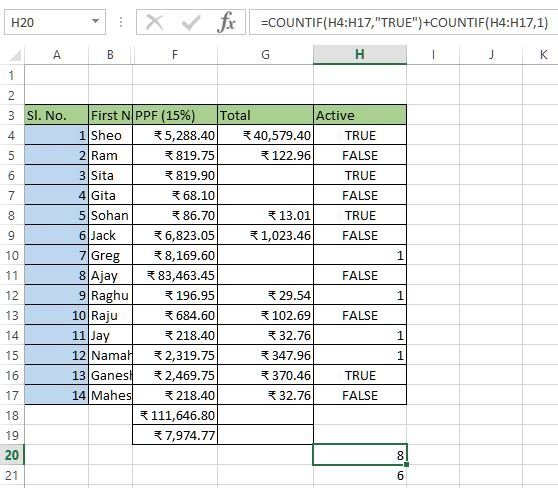# Count Logical and Bit values in Excel

##### How to count logical and mixed logical values in Excel?

In the previous post, we learnt about counting blank and non blank cells in Excel. In this post, we shall learn how to count logical and mixed logical values in MS Excel.

### How to count logical values in Excel?

To count logical values (TRUE/FALSE) in MS Excel, we can use COUNTIF function as explained in previous post. Instead of giving 2nd parameter as "FALSE" (in double quote, we can also give just FALSE as Excel understand these logical values).

So the formula would be '=COUNTIF(H5:H18, FALSE) OR =COUNTIF(H5:H18, "FALSE")'The result is 6.

### How to count mixed logical (also bit) values in Excel?

Let's take example of above data, few records 'Active' field data is TRUE and few are 1 ie. bit value (both are treated as true/yes). If this is the scenario, how to get the correct count of all True/Yes records?

The solution of this problem is to have two COUNTIF functions and add both function's return value.

So the formula would be '=COUNTIF(H4:H17,"TRUE")+COUNTIF(H4:H17,1)'. The 1st part is getting all records count having 'TRUE' and 2nd part is getting all records count having 1. The count of both functions are being added and the result is being displayed in H20 cell.Views: 6293 | Post Order: 18